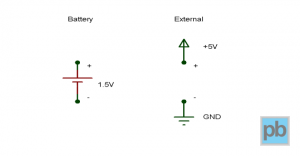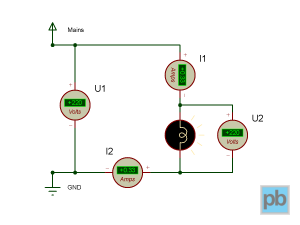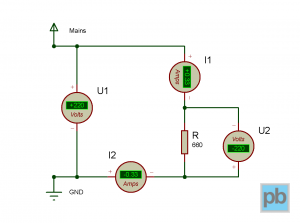# Some basic enlightement

Let’s start with some basic theory, I promise it won’t hurt. First we need to get familiar with some basic concepts like voltage, current, resistance and their relation. Please note that here and throughout this blog, I’m not seeking to provide physically perfect explanations, just a minimal description necessary for me to understand the various phenomenons.

Current

Current is the flow of electricity, which makes everything work, it makes lamps glow, motors revolve and radios play music. Current flows through the wires and electrical components that can conduct electricity, hence they called conductors. In the vast majority of cases, these conductors have subatomic particles in them called electrons, and the flow of current manifests in the movement of these particles. More electrons moving through in a specific timeframe will result in larger electric current. But don’t worry, we don’t have to deal with such microscopic details. Current (I) is measured in amperes (A). Generally we will deal with milliamperes (mA), the thousandth of an ampere (`1 A = 1000 mA`), which is considered small current, while ranges above 10 A are considered large currents.

Voltage

But what makes current begin to flow? I’ll jump right to the answer: voltage does. Voltage has another fancy name, it’s called potential difference. While you wouldn’t call it that way, it helps understanding how it works. Imagine a basketball, this will represent our electron. If you put it on the floor (ground potential), it doesn’t go anywhere. Now if you pick it up, hold it high, then let it go, it doesn’t keep floating int the air but falls down instead. That’s because it was placed to a higher potential, so we have created a potential difference that made the ball start moving.

The way gravitational field affects the basketball is very similar to how electric field affects the electrons. If you create protential difference between two points and connect them with some kind of conductor, current will flow from the point with higher potential to the point with lower one. But how can I create potential difference, you would ask. You have to use a battery or some kind of external power supply like an AC adapter (wall-wart). In circuit diagrams, these power sources are shown as in the following image.Voltage (U) is measured in volts (V). Values below 50 V is considered safe low voltages, flashlight batteries supply 1.5 volts, car batteries supply 12 volts, typical wall adapters supply from 3 to 19 volts. Values above this are dangerous to deal with. Wall sockets (mains) have 110 or 230 volts, while long-distance power lines uses many thousand volts. Generally, higher voltage causes higher current to flow, that’s what makes it dangerous for humans if they touch it with hand.

Power and energy

Power is the product of voltage and current. It describes how much electric energy a device consumes (or to say it a fancy way: dissipates), and how much work can be done with that device. Power (P) is measured in watts (W), the higher the value is the higher power a device has.

The actual amount of electric energy consumed is the product of power and time. Generally, energy (E) is measured in joules (J), but standard household electricity meters show kilowatt-hours (kWh) as units.

Resistance

As it’s name suggests, resistance (R) is a property of electronic devices, it show how much a device obstructs (resists) the flow of current. It is based on the physical characteristics of the device and it is measured in ohms (Ω). A piece of wire for example has a very low resistance (nearly 0 Ω), allowing very large current to flow through it, while a standard lightbulb for example has a resistance around 660 Ω. There are specific electric circuit parts called resistors, which come in various resistance values from less than 1 ohm up to several megaohms, and they are used to limit the amount of current flowing through. The circuit diagram symbol of a resistor is shown on the following image.Getting it all together

There is a correlation between all these properties. Let’s consider the aforementioned lightbulb as an example. It has a tungsten filament that has `R = 660 Ω` resistance because of it’s material and shape. It’s nominal voltage is `U = 230 V`, which will cause `I = U / R = 230 / 660 = 0.348 A` of current to flow through it. The current will make the tungsten filament to heat up and glow. Lower voltage would result in lower current that would cause the lighbulb to glow much more dimmed. This correlation between current, voltage and resistance is called Ohm’s Law (yes, it’s the same Ohm like the resistance measurement unit, named after Georg Ohm).

The power of the lightbulb can be calculated from the voltage and current values by multiplicating them: `P = U × I = 230 × 0.348 = 80 W`. This means that it consumes 80 J of energy every second and if we turn it on for a whole hour, it will consume `E = P × t = 80 W × 1 h = 80 Wh = 0.08 kWh` or `E = P × t = 80 J/s × 3600 s = 288 kJ` of electric energy. Diet enthusiasts can further convert it to 69 calories, but it’s not advisable to eat electricity for breakfast.I created a circuit involving our lightbulb in an electronic circuit design software and ran the simulation. The picture above shows the results (you can click on it to enlarge). In the schematic there is an external power source of 220 V, a 80 W lightbulb, two voltmeters and two ammeters (short for ampere meter). Voltage is always measured between two points, the first voltmeter (`U1`) is placed between the power terminals, the other one (`U2`) measures the voltage between the two terminals of the lightbulb. On the contrary, to measure current, it must flow through the metering device the same way it flows through the lightbulb. Both ammeters (`I1` and `I2`) measure the current flowing through the lightbulb, and thus the entire circuit but they are placed to the different sides of the lightbulb.

If we start the simulation, the lighbulb begins to glow and we can read the values on the meters. It’s no surprise that both voltmeters and both ammeters show the same values, and the values match our calculations above.I also created an equivalent circuit, replacing the lightbulb with a resistor of 660 ohms. I also reversed the polarity (the `+` and `-` ends) of the `U2` voltmeter and the `I2` ammeter, which now show the same values but with the opposite sign. In this circuit, current flows from the higher potential (`+`, `Mains`) towards the lower one (`-`, `GND`), but from the ammeter’s perspective the current flows from its `-` terminal towards its `+` terminal, so it sees reverse current flow which is denoted by the negative sign of the value. Very similarly, the `U2` voltmeter sees that it’s `-` terminal has higher potential that it’s `+` terminal, so the difference between the potentials (aka. voltage) is negative: `0 - 220 V = -220 V`.

Simulation software is very useful for testing an idea of a circuit before building it. Doing so you can avoid dangerous situations which could harm to you or damage your devices or equipment. Nevertheless, having such a tool, you can bypass lots of math calculations with a trial-and-error method.

Summary

I hope I could shed a light on the basic principles of electronics. I’ve created a small cheat sheet with the aforementioned physics terms and their relations:

```Current (I)          I = U / R        I = P / U
1 A
Voltage (U)          U = I × R        U = P / I
1 V
Resistance (R)       R = U / I
1 Ω
Power (P)            P = U × I
1 W = 1 J/s
Energy (E)           E = P × t
1 J = 1 Ws
1 kWh = 3600 kWs = 3600000 Ws = 3600000 J```